# Blueberries

5 children collect 4 liters of blueberries in 1.5 hours.
a) How many minutes do 3 children take 2 liters of blueberries?
b) How many liters of blueberries will be taken by 8 children in 3 hours?

Result

t =  75 min
V =  12.8 l

#### Solution:

$h = \dfrac{ 4 }{ 1.5 \cdot \ 5 } = \dfrac{ 8 }{ 15 } \doteq 0.5333 \ l/h/h \ \\ \ \\ t_{ 1 } = \dfrac{ 2 }{ 3 \cdot \ h } = \dfrac{ 2 }{ 3 \cdot \ 0.5333 } = \dfrac{ 5 }{ 4 } = 1.25 \ h \ \\ \ \\ t = t_{ 1 } \rightarrow min = t_{ 1 } \cdot \ 60 \ min = 75 \ min = 75 \ \text { min }$
$V = 8 \cdot \ 3 \cdot \ h = 8 \cdot \ 3 \cdot \ 0.5333 = \dfrac{ 64 }{ 5 } = 12.8 = 12.8 \ \text { l }$

Our examples were largely sent or created by pupils and students themselves. Therefore, we would be pleased if you could send us any errors you found, spelling mistakes, or rephasing the example. Thank you!

Leave us a comment of this math problem and its solution (i.e. if it is still somewhat unclear...):Be the first to comment!#### Following knowledge from mathematics are needed to solve this word math problem:

Need help calculate sum, simplify or multiply fractions? Try our fraction calculator.

## Next similar math problems:

1. Hectoliters of waterThe pool has a total of 126 hectoliters of water. The first pump draws 2.1 liters of water per second. A second pump pumps 3.5 liters of water per second. How long will it take both pumps to drain four-fifths of the water at the same time?
2. Liters od milkThe cylinder-shaped container contains 80 liters of milk. Milk level is 45 cm. How much milk will in the container, if level raise to height 72 cm?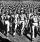Road repair took 10 days for 36 workers if they worked 9 hours a day. How many days take repair same road 37 workers if they work 8 per day?
4. Assembly parts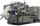Nine machines produce 1,800 parts on nine machines. How many hours will it produce 2 100 parts on seven such machines?
5. Seven workers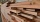Seven workers clear the glade in 22 hours. How many workers would need to be done in 8 hours?
6. Two workers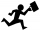One worker needs 40 hours to do a job, and the second would do it in 30 hours. They worked together for several hours, then the second was recalled, and the first completed the job itself in 5 hours. How many hours did they work together, and how much did.
7. Two pumps togetherThe first pump will fill the tank itself in 3 hours and the second one in 6 hours. How many hours will the tank be full if both pumps are worked at the same time?
8. Six studentsTwo pupils painted the class in four hours. How long will it take for six pupils?
9. Trucks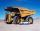Truck take you the some amount of clay in 30 hours. Second truck in 40 hours. Trucks carted the clay away some time together then the second truck broke down and the first one completed the job in five hours. What time worked together?
10. Workers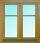Group of workers washing half of the windows of the building in two hours. How long will take half the workers wash all the windows of the building?
11. Apprentice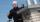Worker and apprentice execute the work for 6 hours. Worker performs himself in 10 hours. How long do the work of an apprentice?
12. 4 pipes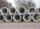The tank flows out by 4 pipes in 6 hours 120 hl water. How much water flows out of 5 pipes of the same diameter in 14 hours?
13. Two typists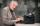There are two typists who are rewriting the material 814 pages. First can it handle rewrite yourself for 24 days; the second 12 days. First typist wrote material yourself 4 days rest rewrites yourself second typist. How many days will it take rewriting alt
14. The tank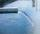The tank had 9 inflows to be filled in 21 days. After 9 days, 3 trips out. How many days did the remaining 6 tributaries fill the tank?
15. The sum 4The sum of Robin's age is 45. Seven years ago, Robin was 16 years more than one half as old as Bruno then. How old is Bruno?
16. Six workersSix workers planned to repair the roof in 5 days. After two days, two workers get sick. How long will the remaining workers complete the roof at the same rate of work?
17. Four painters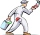The company sent four painters to paint the school. They should be done in 12 days. After three days, one painter got ill. How long will the remaining painters paint the school?